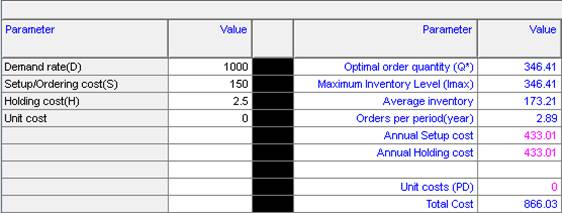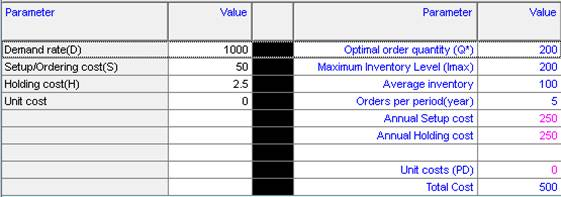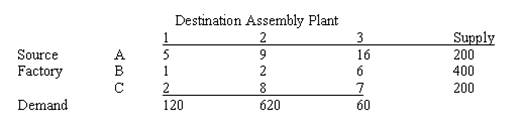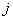# Operations Management Help

 We offer quality and prompt Operations Management Homework Help We can help you with any kind of Operations Management assignments, homeworks, tests, exams, at any level, including topics such as Forecasting, PERT, Aggregate Planning, Waiting Lines, Network Problems, Linear Programming and Transportation Problems, Inventory, Quality Control and much more. We can help you with different software packages, such as Excel QM, POM, @RISK, Crystal Ball and The Management Scientist. Our service is convenient, confidential and efficient. Our rate starts $35/hour. We will send you a Free Quote in hours. Quick turnaround! • Forecasting • Moving Averages • Exponential Smoothing • Linear Trends • Seasonal Components • Statistical Process Control • Control Charts • X-bar, R-bar, p-bar, C-bar charts • Process Capability • Decision Theory • Maximax and Maximin • Minimax Regret • Expected Monetary Value • Expected Value of perfect information (EVPI) • Expected Value of sample information (EVSI) • Decision Trees • Utility • Location Strategies • Factor Rating • Center of Gravity • Transportation Model • Layout Strategy • Fix-position layout • Processed Oriented layout • Office layout • Warehouse layout • Forecasting • Inventory Models • A-B-C analysis • Economic Order Quantity (EOQ) • Economic Production Quantity (EPQ) • Quantity Discount Models • One-Period Model • Just in Time systems (JIT) • PERT • Single Estimate • Triple Estimate • Cost Crashing • Critical Paths • Gantt-Charts • Aggregate Scheduling • Demand Chase • Mixed Strategy • Material Requirement Planning (MRP) • Bills of material • Lot-for-lot • Linear Programming • Linear Programming • Transportation Problems • Transshipment • Integer Programming • Assignment Problems • Network Problems • Waiting-Line models • Queues • Single-Channel and Multi-Channel models • Simulation • Simulations in Excel • Monte Carlo Methods ## Why we can help with your Operations Management? #### Year of Experience We have been online for more than 10 years, we have worked with thousands of customers who have been able to appreciate the quality of our work #### Operations Management Expertise Operations Management can be a tough subject due to the many concepts that need to be mastered and the number of formulas a student need to master in order to be in good standing. Our tutors are the right experts to help you with your homework or anything academic related with Operations Management #### Step-by-Step Solutions Our tutors provide detailed, step-by-step solutions, and we put a lot of care in double checking our calculations #### Free Quote You can e-mail us your problems 24x7. We will send a free quote ASAP #### Very Competitive Prices We try to accommodate to all budgets. No job is too big or too small with us. We make our best to accommodate to our customers' needs #### We take pride of our work We do our work with care. We are experts and we take pride in what we do. Our main objective is our customers' complete satisfaction. We take great care in paying attention to all the requirements and details, with the purpose of fulfilling work of the highest quality ## and more... #### Prices Prices start at$35 per hour, depending on the complexity of the work and the turnaround time

You can e-mail us your problems for a free quote.

Question: The costs of placing an order is $150. It is estimated that 1,000 units will be used in the next 12 months. The carrying cost per unit per month is$2.50.

a. Compute the optimum order size

b. Now suppose that the company could lower the ordering cost to $50; the cost of the effort to accomplish this change is$1,000. Assume the product will be sold only for the next two years. Compute the new optimal order quantity if the lower ordering cost were implemented, and determine if the company should invest $1,000 in the ordering-cost reduction program. Solution: (a) The following is obtained:Hence, the optimal order quantity is $EOQ=\sqrt{\frac{2DS}{H}}=\sqrt{\frac{2\times 1000\times 150}{2.5}}=346.41$ The total inventory cost is TC =$866.03.

(b) IF the setup cost was reduced to $50, we would getIn this case, the optimal order quantity is EOQ = 200, and the total inventory cost is$500. The savings in cost for 2 years operation are (866.03 – 500)*2 =$732.06, which is less than$1000 (the cost of reducing the setup cost), and hence, the ordering-cost reduction program should not be pursued.

Question: A logistics specialist for Wiethoff Inc. must distribute cases of parts from 3 factories to 3 assembly plants. The monthly supplies and demands, along with the per-case transportation costs are:What are the total monthly transportation costs for the optimal solution?

Solution: We have 9 variables $${{x}_{ij}}$$, where $${{x}_{ij}}$$ represents the amount that is sent from factoryto assembly plant. For convenience of notation, we name the factories A, B and C as 1, 2 and 3.

The problem is written as

\begin{align} & \text{Minimize }\sum\limits_{i,j=1}^{3}{{{C}_{ij}}{{X}_{ij}}} \\ & \text{subject to }{{X}_{11}}+{{X}_{12}}+{{X}_{13}}\le 200 \\ & \text{ }{{X}_{21}}+{{X}_{22}}+{{X}_{23}}\le 400 \\ & \text{ }{{X}_{31}}+{{X}_{32}}+{{X}_{33}}\le 200 \\ & \text{ }{{X}_{11}}+{{X}_{21}}+{{X}_{31}}=120 \\ & \text{ }{{X}_{12}}+{{X}_{22}}+{{X}_{32}}=620 \\ & \text{ }{{X}_{13}}+{{X}_{23}}+{{X}_{33}}=60 \\ & \text{ }{{X}_{ij}}\ge 0 \\ \end{align}

We know solve this problem using Excel’s solver. The output is shown below:

 Plants Variables 1 2 3 1 2 3 A 5 9 16 A 0 200 0 Factories B 1 2 6 B 0 400 0 C 2 8 7 C 120 20 60 1 2 3 $${{\mathbf{c}}_{ij}}{{\mathbf{x}}_{ij}}$$ 1 0 1800 0 2 0 800 0 Objective 3420 3 240 160 420 Restrictions Supply Slack 1 0 200 Slack 2 0 400 Slack 3 0 200 Demand Slack 4 0 Slack 5 0 Slack 6 0

This means that 120 cases of parts are sent from factory C to Plant 1. The total monthly transportation costs are \$3,420.